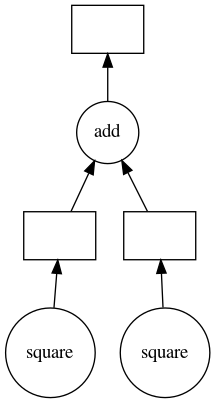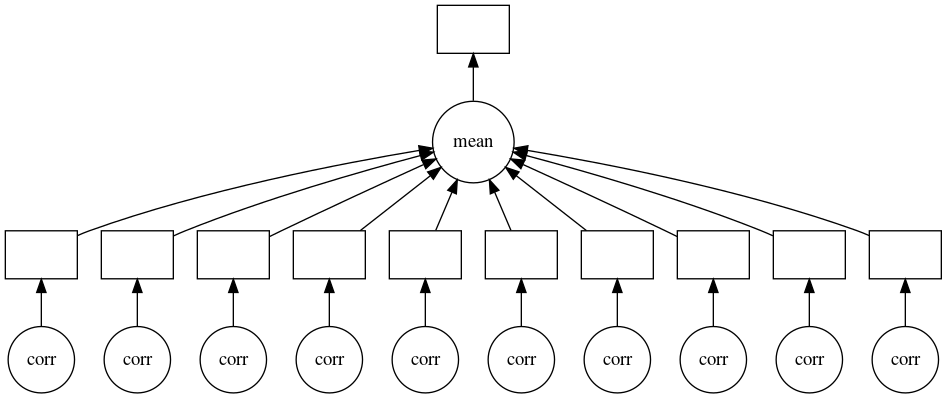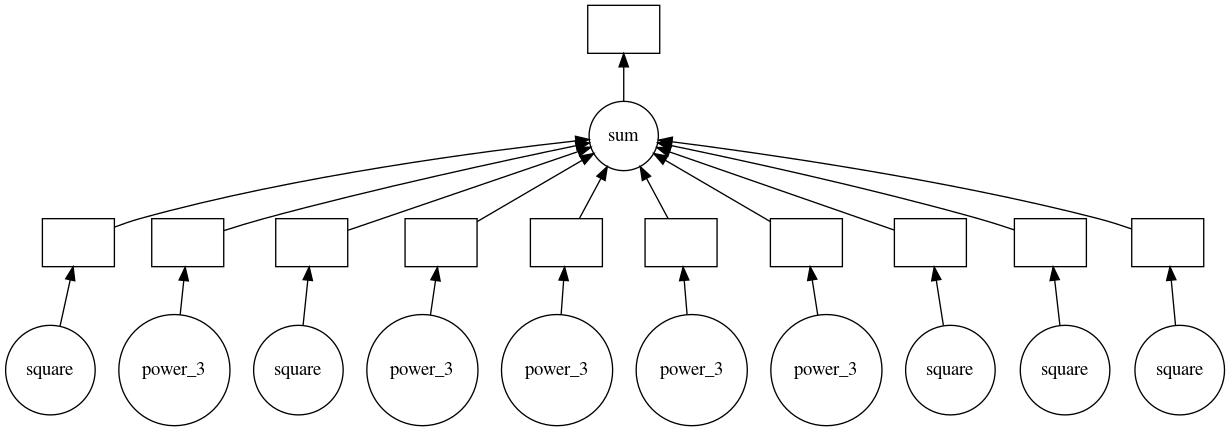Updated On : May-10,2020 Time Investment : ~30 mins

# dask.delayed - Parallel Computing in Python¶

## Introduction ¶

Dask is a python library that provides a list of APIs for performing the computation in parallel on a single computer as well as a cluster of computers. We have already created tutorial on dask.bag API which is spark like API for parallelizing big data. Dask has other APIs like `dask.delayed`, `dask.dataframe`, `dask.array` and `dask.distributed` whose introduction we have covered in the previous tutorial. We suggest that you go through that tutorial to get basic information about the dask. We'll be specifically concentrating on `dask.delayed` API as a part of this tutorial.

The `dask.delayed` provides a very flexible API which lets us parallelize our python functions. It's very suitable for problems when it doesn't involve data structures like `dask.array` or `dask.dataframe`. The API of dask.delayed is simplified which lets us parallelize our python code very easily.

### Directed Acyclic Graph (DAG) of Execution ¶

The `dask.delayed` API is used to convert normal function to lazy function. When a function is converted from normal to lazy, it prevents function to execute immediately. Instead, its execution is delayed in the future. Dask can easily run these lazy functions in parallel.

The `dask.delayed` API keeps on creating a directed acyclic graph of these lazy functions in the background. This graph keeps track of the execution sequence of these lazy functions which helps it understand which parts can be executed in parallel. When we evaluate this graph by calling `compute()` method of dask.delayed API then it evaluates all functions by running them in parallel. We'll be visualizing graphs created by dask when explaining the usage of `dask.delayed` API.

This ends our small introduction of `dask.delayed` API. We'll be explaining its usage with few examples below.

So without further delay, let’s get started with the coding part.

We'll first import all the necessary libraries for a tutorial.

```import dask

import numpy as np
import pandas as pd

import time
import sys
import os

print("Python Version : ", sys.version)
```
```Python Version :  3.7.3 (default, Mar 27 2019, 22:11:17)
[GCC 7.3.0]
```

## Common Way of Using `dask.delayed` API ¶

We can easily parallelize our normal python code using dask by following list of below steps:

• Find out the list of time-consuming functions in code that are getting executed more than once.
• Convert a list of time-consuming functions from `normal` to `lazy` by using `dask.delayed`. All functions calling these lazy functions will also become lazy.
• Execute last lazy function. There is generally two scenarios of this.
• `Scenario 1:` You only need to execute one final function which calls a list of lazy functions. If this is the scenario then you can call compute() on final lazy function and it'll run all lazy functions it depends on in parallel.
• `Scenario 2:` If there are more than one final lazy functions then we can use `compute()` method of `dask`. We need to pass all lazy functions to this method and it'll run all of them in parallel and returns results for all of them.

We'll be explaining these steps below with few examples to make things clear. We'll be creating normal python functions which takes time to execute normally but runs faster when parallelized using dask.

### Optional Step: Start Dask Client to Analyze Results¶

Dask provides an optional dashboard which we can utilize to see the performance of operations getting performed in parallel. This step is optional if you don't want to analyze results but it can be very useful to debug things.

```from dask.distributed import Client
client
```

### Cluster

• Workers: 4
• Cores: 16
• Memory: 3.95 GB

We suggest that you pass `n_workers` same as a number of cores on your PC and `threads_per_worker` as 1 if you are running code on local.

You can open the URL mentioned next to the dashboard. It'll have a list of tabs that provides information like running time of the task, CPU usage, memory usage, directed graphs, etc. All this can be useful for analyzing parallel execution of tasks. You'll be able to see the whole directed acyclic graph of lazy functions as well in the `Graph` section of the dashboard. They will be all marked `blue` once that particular lazy function node has completed execution. If your function has many lazy functions then you'll be able to see changing their values in real-time to `blue` when they complete.

### Example 1 ¶

Below we have created a simple python example which takes the square of two numbers and adds them. We have created a function for getting square of numbers. We have artificially added sleep time of 1 second to function to mimic a real-life situations where functions can take time to execute. We are calling this function 2 times to get a square of 2 different numbers before adding them. As per below pure python code, it executes `square` function sequentially which results in taking 2 seconds for code to complete.

```%%time

def square(x):
time.sleep(1)
return x*x

x_sq = square(3)
y_sq = square(4)

res = x_sq + y_sq
res
```
```CPU times: user 149 ms, sys: 14.4 ms, total: 163 ms
Wall time: 2 s
```
`25`

We'll now try to convert the above example to the dask version so that it can run fast using parallelization. From the above code, we can easily notice that `square` function takes time to execute. We can even notice that each execution of the square is totally independent of any other execution of the same function which makes it ideal for parallel execution.

We'll convert each square function execution to `lazy` function by calling `delayed` on them as explained below.

```%%time

x_sq = delayed(square)(3)
y_sq = delayed(square)(4)

res = x_sq + y_sq
res
```
```CPU times: user 2.89 ms, sys: 0 ns, total: 2.89 ms
Wall time: 2.66 ms
```
`Delayed('add-efc08ea624c87229d28849298d3bc486')`

We can notice from above cell execution that it executed immediately. It's because of dask.delayed API has just converted normal square execution to lazy execution and has created an execution graph as of now. But it has not actually executed functions. We can see that the return type of res is `Delayed` object instead of the result.

We can make dask execute code in parallel by calling `compute()` method on the final `res` object. It'll evaluate the graph and execute a square function in parallel.

```%time res.compute()
```
```CPU times: user 110 ms, sys: 15.8 ms, total: 125 ms
Wall time: 1.04 s
```
`25`

We can notice from the above cell that it takes less time to compute the sum of squares using `dask.delayed` API.

We can even visualize DAG created by dask by calling `visualize()` method on the final `res` object.

```res.visualize()
```### Example 2 ¶

Below we are explaining another usage of dask.delayed API. Here we are creating an array of random numbers of size 10x1000. We then want to compute the correlation between the first row of the array and all other rows. We have implemented this solution as a loop. We also have designed the function `corr` which calculates correlation. We have added a time delay of 1 second into function execution to mimic real-life situations.

```%%time

x = np.random.rand(10,1000)

def corr(x, y):
time.sleep(1)
return np.corrcoef(x, y)

correlations = []

for row in x:
correlations.append(corr(x, row))

avg_corr = np.mean(correlations)
avg_corr
```
```CPU times: user 922 ms, sys: 117 ms, total: 1.04 s
Wall time: 10 s
```
`0.07951399677238377`

We can notice that the above code takes 10 seconds for execution because there are 10 rows in an array. We are computing a correlation between the first row and all other rows, all of which take 1 second.

We are below converting the above code to dask delayed code so that it runs in parallel. We have used delayed at two places this time. First on `corr()` function and then on `np.mean()` functions.

```%%time

def corr(x, y):
time.sleep(1)
return np.corrcoef(x, y)

correlations = []

for row in x:
correlations.append(delayed(corr)(x, row))

avg_corr = delayed(np.mean)(correlations)
avg_corr
```
```CPU times: user 3.13 ms, sys: 0 ns, total: 3.13 ms
Wall time: 3.13 ms
```
`Delayed('mean-e8b81f7e-4ca6-4a60-bcd3-ffbfec1d4fef')`

Like the previous example, we can notice that the above code with lazy functions executes fast because actual execution of code has not happened yet.

We'll not call `compute()` on final `avg_corr` variable to complete execution.

```%time avg_corr.compute()
```
```CPU times: user 146 ms, sys: 14.7 ms, total: 161 ms
Wall time: 1.23 s
```
`0.07951399677238377`

We can notice that it completes execution is quite less time compared to normal sequential execution.

Below we are visualizing the execution graph of the above code.

```avg_corr.visualize()
```### Example 3 ¶

Below we are demonstrating usage of `dask.delayed` API using the third example. We are first looping through number 1-10 taking the square of the number if the number is divisible by 2 else taking power 3 of number. We have defined function for taking a square and power 3 of numbers. We have also added time delay in function so that it looks like a real-life situation.

```%%time

def square(x):
time.sleep(1)
return x*x

def power_3(x):
time.sleep(1)
return x*x*x

final_list = []
for i in range(1,11):
if i%2 == 0:
final_list.append(square(i))
else:
final_list.append(power_3(i))

res = sum(final_list)
res
```
```CPU times: user 896 ms, sys: 131 ms, total: 1.03 s
Wall time: 10 s
```
`1445`

We can see above that when we run above loop in parallel then it takes 10 seconds for it to complete. We can easily convert this code using `dask.delayed` as explained below. This example demonstrates how conditional functions can also be parallelized using dask.

We have made use of `@delayed` annotation this time instead of wrapping calls of function like previous examples. We can also use the `annotation` above function rather than wrapping each call of function into `delayed`.

```%%time

@delayed
def square(x):
time.sleep(1)
return x*x

@delayed
def power_3(x):
time.sleep(1)
return x*x*x

final_list = []
for i in range(1,11):
if i%2 == 0:
final_list.append(square(i))
else:
final_list.append(power_3(i))

res = delayed(sum)(final_list)
res
```
```CPU times: user 3.09 ms, sys: 3.31 ms, total: 6.39 ms
Wall time: 4.22 ms
```
`Delayed('sum-37687f32-c4a0-4c43-a69f-33900eda0570')`
```%time res.compute()
```
```CPU times: user 134 ms, sys: 16.4 ms, total: 150 ms
Wall time: 1.06 s
```
`1445`
```res.visualize()
```### Example 4 ¶

As a part of our 4th example, we'll be working with some files. We are creating 30 CSV files for 30 days of temperature data where the temperature is recorded every 5 seconds. We are first creating CSV files into a separate folder for each day. Each CSV file will have 17280 temperature values for that day recorded at every 5 seconds. We are filling temperature data with a random temperature between 1-50.

```if not os.path.exists("temp_dataframes"):
os.mkdir("temp_dataframes")

for i in range(1,31):
dates= pd.date_range(start="1-%d-2020"%i, end="1-%d-2020"%(i+1), freq="5S")[:-1]
temp_df = pd.DataFrame(np.random.randint(1,50, 17280), index=dates, columns=["Temp"])
temp_df.to_csv("temp_dataframes/1-%d-2020.csv"%i)
```

Below we are looping through temperature file of each day, reading it, taking an average of that day, and adding it to list. We are then taking an average of all days combined. Our main aim of this example was to explain the usage of `dask.delayed` API working with files.

```%%time

def calc_mean(f_name):
return df.mean().values

avg_temp_per_day = []
for i in range(1,31):
avg_temp_per_day.append(calc_mean("temp_dataframes/1-%d-2020.csv"%i))

avg_temp = np.mean(avg_temp_per_day)
avg_temp
```
```CPU times: user 11.7 s, sys: 136 ms, total: 11.9 s
Wall time: 11.7 s
```
`24.999012345679013`

We can see that it takes nearly 12 seconds for the above code to complete.

We have converted it to dask code below which completes in nearly 3 seconds.

```%%time

@delayed
def calc_mean(f_name):
return df.mean().values

avg_temp_per_day = []
for i in range(1,31):
avg_temp_per_day.append(calc_mean("temp_dataframes/1-%d-2020.csv"%i))

avg_temp = delayed(np.mean)(avg_temp_per_day)
avg_temp
```
```CPU times: user 0 ns, sys: 2.88 ms, total: 2.88 ms
Wall time: 2.23 ms
```
`Delayed('mean-c3488a5c-7a16-4615-99f3-f959198c748f')`
```%time avg_temp.compute()
```
```CPU times: user 141 ms, sys: 29.1 ms, total: 170 ms
Wall time: 3.95 s
```
`24.999012345679013`

### Example 5 ¶

Our fifth and last example is designed to explain the usage of `compute()` method available directly from dask which takes an input list of lazy objects and can evaluate them.

Below we are looping through 1-10, creating an array of random numbers of size 100 between 1-100. We are then taking sum and count of numbers divisible by 2. We add all sums and counts to an array. We then sum the array of sums and divide it by sum of counts array to get average. We have added time delay to function so that it takes time to mimicking real-life situations.

```%%time

def total_func(x):
time.sleep(0.5)
return sum([i for i in x if i%2==0])

def count_func(x):
time.sleep(0.5)
return len([i for i in x if i%2==0])

totals, counts = [], []
for i in range(10):
x = np.random.randint(1,100,1000)
total = total_func(x)
count = count_func(x)

totals.append(total)
counts.append(count)

avg_num = sum(totals) / sum(counts)
avg_num
```
```CPU times: user 963 ms, sys: 180 ms, total: 1.14 s
Wall time: 10.1 s
```
`49.85420861860653`

Below we have converted the above example to `dask.delayed` version. We have annotated both functions with `@delayed` annotation. We have evaluated both `totals_orig` and `counts_orig` array which are list of delayed objects using `compute()` function. We can see that this one takes nearly 1 second to complete compared to the previous version.

```%%time

@delayed
def total_func(x):
time.sleep(0.5)
return sum([i for i in x if i%2==0])

@delayed
def count_func(x):
time.sleep(0.5)
return len([i for i in x if i%2==0])

totals_orig, counts_orig = [], []
for i in range(10):
x = np.random.randint(1,100,1000)
total = total_func(x)
count = count_func(x)

totals_orig.append(total)
counts_orig.append(count)

totals, counts = compute(totals_orig, counts_orig)
avg_num = sum(totals) / sum(counts)
avg_num
```
```CPU times: user 168 ms, sys: 28.8 ms, total: 196 ms
Wall time: 1.09 s
```
`49.56845298281092`
```totals_orig
```
```[Delayed('total_func-85fc3673-c004-45d1-81f8-f08509e7d79a'),
Delayed('total_func-10b5ebca-d6f5-45f7-b2d1-882c7391fd7e'),
Delayed('total_func-6c44bd15-fc20-4682-82cb-6446a414eab7'),
Delayed('total_func-e50378bb-c06c-414e-b57e-71d123aff579'),
Delayed('total_func-6ce847de-c7b4-4b08-9207-751a6733b8ee'),
Delayed('total_func-b512e024-9c64-426b-8036-09ba355daa85'),
Delayed('total_func-6dd12b7c-9a4c-44b5-8a52-a803d44432f3'),
Delayed('total_func-b6ac83a0-c108-4d20-8c68-5ed3ca982d2e')]```
```counts_orig
```
```[Delayed('count_func-42da9b82-ca2f-4294-8b0e-5013bd30f3d9'),
Delayed('count_func-c8124297-4dea-432f-8e0a-e709bc8294a2'),
Delayed('count_func-de8a79e4-85c7-4d39-942c-47890c647b6b'),
Delayed('count_func-6d64ef92-d561-42ca-a507-902d01906e99'),
Delayed('count_func-afde8147-4294-4d7b-af24-829933747b0d'),
Delayed('count_func-03b89eae-bc9c-4456-9f58-3643c53bfbd8'),
Delayed('count_func-0155b304-7f18-4016-93af-4cbf9e84a867'),
Delayed('count_func-951b32a7-7860-49f1-af7c-258a8ce1aaf7')]```

This ends our small tutorial explaining the usage of dask.delayed API for parallelizing python code. Please feel free to let us know your views in the comments section.

Sunny Solanki

## Comfortable Learning through Video Tutorials?

If you are more comfortable learning through video tutorials then we would recommend that you subscribe to our YouTube channel.

## Stuck Somewhere? Need Help with Coding? Have Doubts About the Topic/Code?

When going through coding examples, it's quite common to have doubts and errors.

If you have doubts about some code examples or are stuck somewhere when trying our code, send us an email at coderzcolumn07@gmail.com. We'll help you or point you in the direction where you can find a solution to your problem.

You can even send us a mail if you are trying something new and need guidance regarding coding. We'll try to respond as soon as possible.

## Want to Share Your Views? Have Any Suggestions?

If you want to

• provide some suggestions on topic